# 1、盛水最多的容器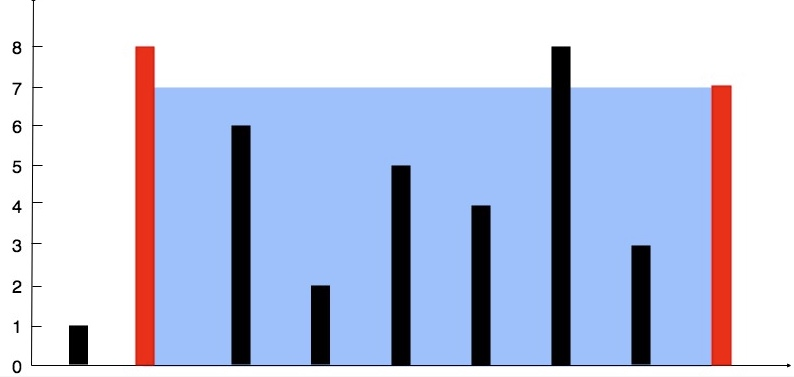输入: [1,8,6,2,5,4,8,3,7]



## 1.1、解题思路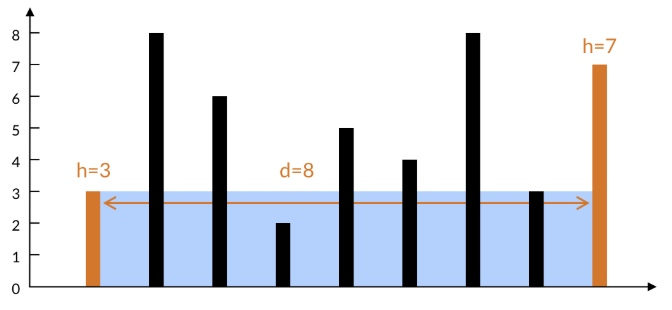1、左边柱子较短，决定了水的高度为 3。如果移动左边的柱子，新的水面高度不确定，但是一定不会超过右边的柱子高度 7。

2、如果移动右边的柱子，新的水面高度一定不会超过左边的柱子高度3，也就是不会超过现在的水面高度。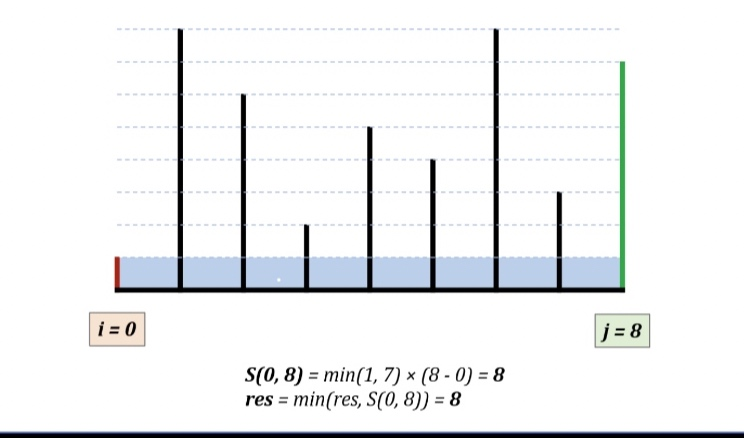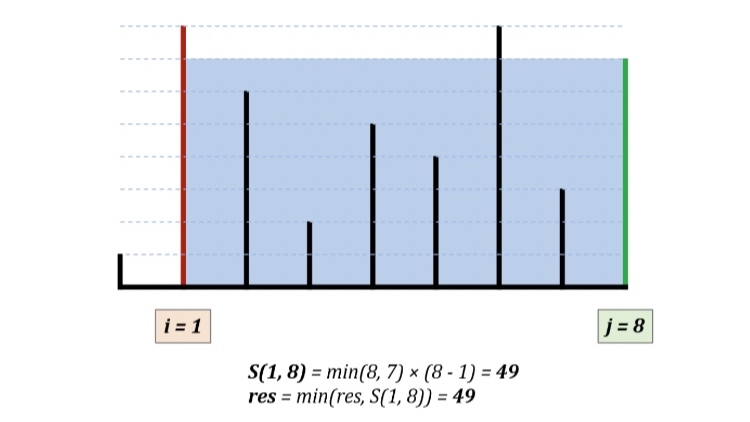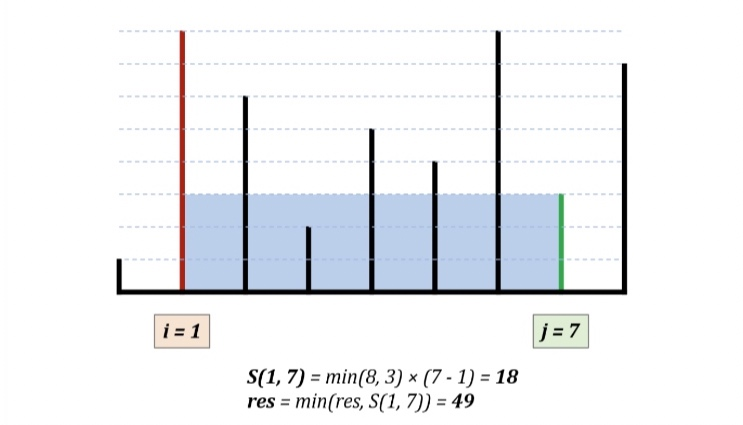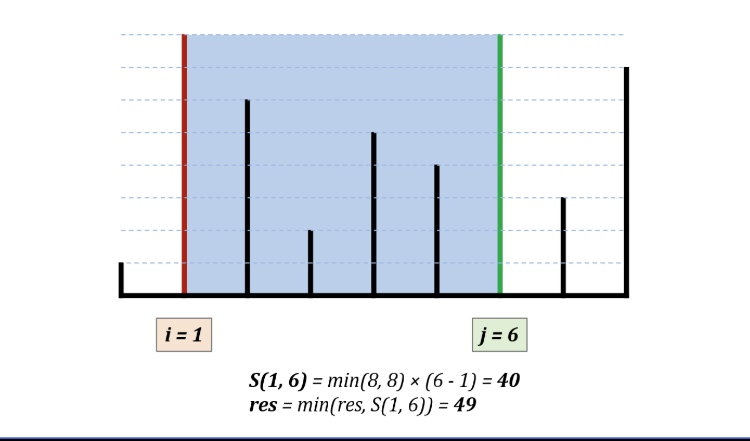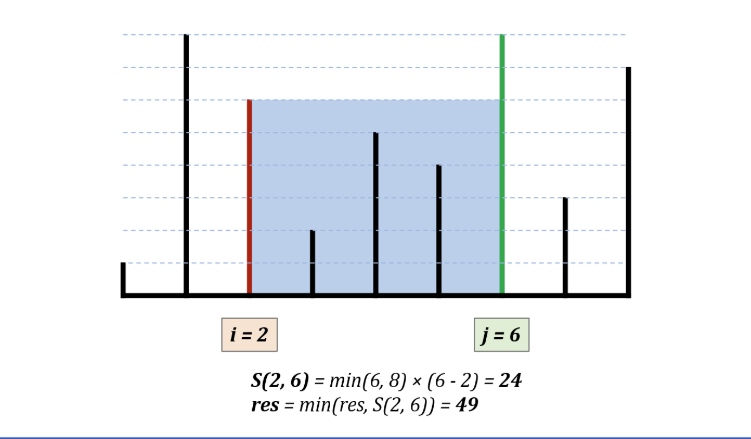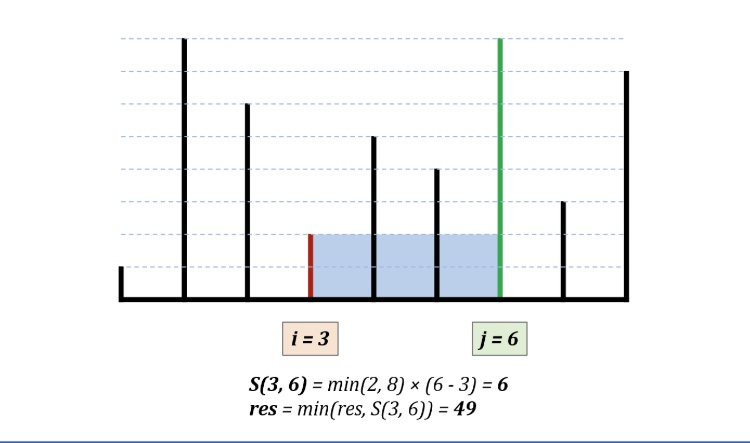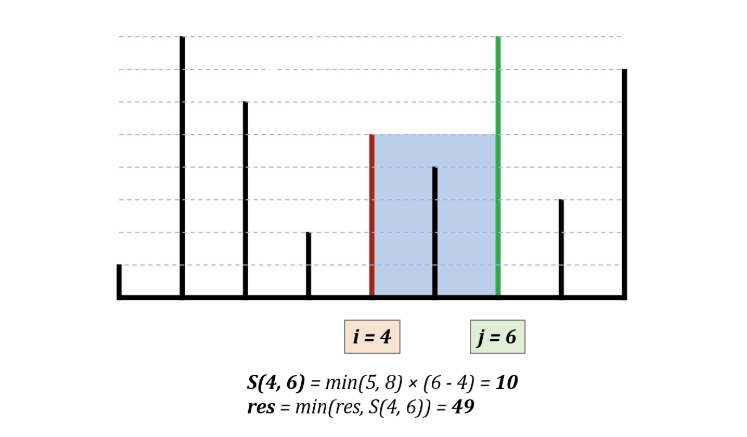## 1.2、算法



public int maxArea(int[] height) {
//指针
int i = 0 ,j = height.length -1 ;
//j和i之间的长度
int length = 0 ;
int max = 0 ;
int minHeight = 0 ;
while (i < j){
length = j - i ;
if (height[i] < height[j]){
minHeight = height[i] ;
i++ ;
}else {
minHeight = height[j];
j-- ;
}
max = Math.max(max, length * minHeight);
}
return max ;
}



## 1.3、测试


@Test
public void test(){
int[] height = new int[]{1,8,6,2,5,4,8,3,7};
System.out.println( maxArea(height));
}

49